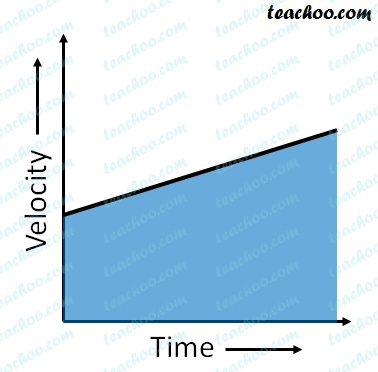## What is the quantity which is measured by the area occupied below the velocity-time graph?Area under v-t graph will be multiplication of Velocity and Time

Since Displacement = Velocity x Time

The area under velocity-time graph gives displacement.

Subscribe to our Youtube Channel - https://you.tube/teachoo

1. Class 9
2. Chapter 8 Class 9 - Motion
3. Questions from Inside the chapter

Questions from Inside the chapter

Class 9
Chapter 8 Class 9 - Motion